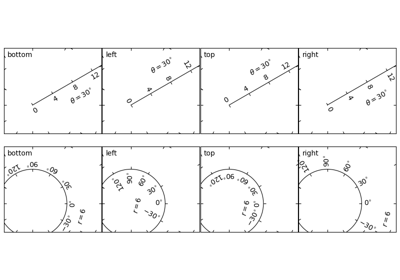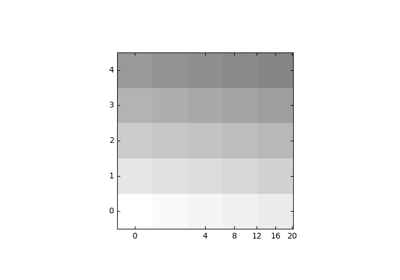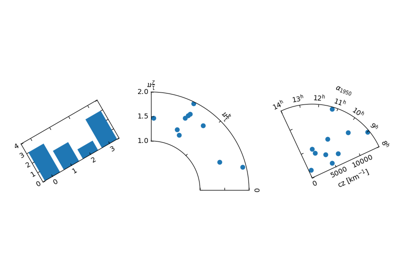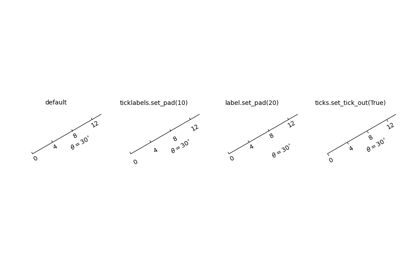# mpl_toolkits.axisartist.grid_finder.MaxNLocator¶

class mpl_toolkits.axisartist.grid_finder.MaxNLocator(nbins=10, steps=None, trim=True, integer=False, symmetric=False, prune=None)[source]
Parameters: nbinsint or 'auto', optional, default: 10Maximum number of intervals; one less than max number of ticks. If the string 'auto', the number of bins will be automatically determined based on the length of the axis. stepsarray-like, optionalSequence of nice numbers starting with 1 and ending with 10; e.g., [1, 2, 4, 5, 10], where the values are acceptable tick multiples. i.e. for the example, 20, 40, 60 would be an acceptable set of ticks, as would 0.4, 0.6, 0.8, because they are multiples of 2. However, 30, 60, 90 would not be allowed because 3 does not appear in the list of steps. integerbool, optional, default: FalseIf True, ticks will take only integer values, provided at least min_n_ticks integers are found within the view limits. symmetricbool, optional, default: FalseIf True, autoscaling will result in a range symmetric about zero. prune{'lower', 'upper', 'both', None}, optional, default: NoneRemove edge ticks -- useful for stacked or ganged plots where the upper tick of one axes overlaps with the lower tick of the axes above it, primarily when rcParams["axes.autolimit_mode"] (default: 'data') is 'round_numbers'. If prune=='lower', the smallest tick will be removed. If prune == 'upper', the largest tick will be removed. If prune == 'both', the largest and smallest ticks will be removed. If prune == None, no ticks will be removed. min_n_ticksint, optional, default: 2Relax nbins and integer constraints if necessary to obtain this minimum number of ticks.
set_factor(self, f)[source]

## Examples using mpl_toolkits.axisartist.grid_finder.MaxNLocator¶